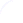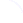Search
 Submission Procedure share: |Follow usavailable in:   PDF (476 kB) PS (1 MB)

get:
 Similar Docs BibTeX Write a comment
get:
 Links into Future

DOI:   10.3217/jucs-016-05-0622

### NP-completeness and FPT Results for Rectilinear Covering Problems

Vladimir Estivill-Castro (Griffith University, Australia)

Apichat Heednacram (Griffith University, Australia)

Francis Suraweera (Griffith University, Australia)

Abstract: This paper discusses three rectilinear (that is, axis-parallel) covering problems in d dimensions and their variants. The first problem is the RECTILINEAR LINE COVER where the inputs are n points in ℝd and a positive integer k, and we are asked to answer if we can cover these n points with at most k lines where these lines are restricted to be axis parallel. We show that this problem has efficient fixed-parameter tractable (FPT) algorithms. The second problem is the RECTILINEAR k-LINKS SPANNING PATH PROBLEM where the inputs are also n points in ℝd and a positive integer k but here we are asked to answer if there is a piecewise linear path through these n points having at most k line-segments (links) where these line-segments are axisparallel. We prove that this second problem is FPT under the assumption that no two line-segments share the same line. The third problem is the RECTILINEAR HYPERPLANE COVER problem and we are asked to cover a set of n points in d dimensions with k axis-parallel hyperplanes of d - 1 dimensions. We also demonstrate this has an FPT-algorithm. Previous to the results above, only conjectures were enunciated over several years on the NP-completeness of the RECTILINEAR MINIMUM LINK TRAVELING SALESMAN PROBLEM, the MINIMUM LINK SPANNING PATH PROBLEM and the RECTILINEAR HYPERPLANE COVER. We provide the proof that the RECTILINEAR MINIMUM LINK TRAVELING SALESMAN PROBLEM and the RECTILINEAR MINIMUM LINK SPANNING PATH PROBLEM are NP-complete by a reduction from the ONE-IN-THREE 3-SAT problem. The NP-completeness of the RECTILINEAR HYPERPLANE COVER problem is proved by a reduction from 3-SAT. This suggests dealing with the intractability just discovered with fixed-parameter tractability. Moreover, if we extend our problems to a finite set of orientations, our approach proves these problems remain FPT.

Keywords: computational geometry, parameterized complexity, restricted orientations

Categories: F.1, F.2, F.2.2